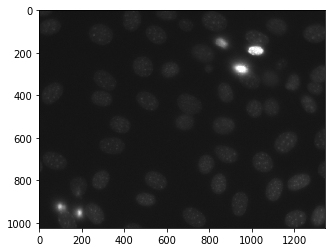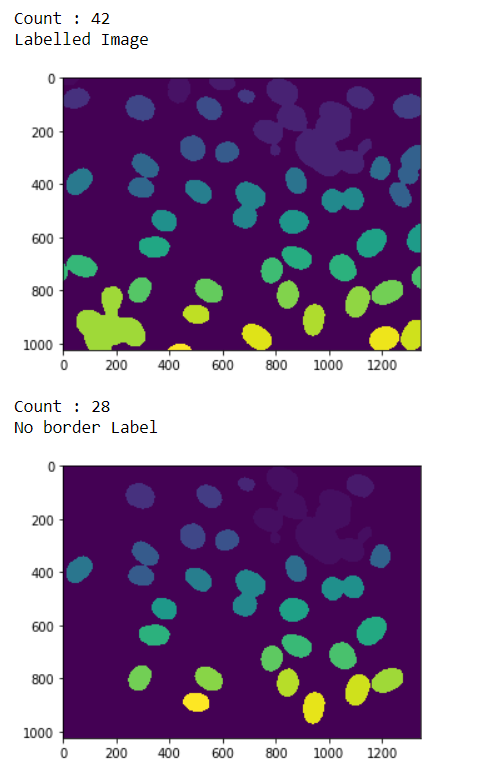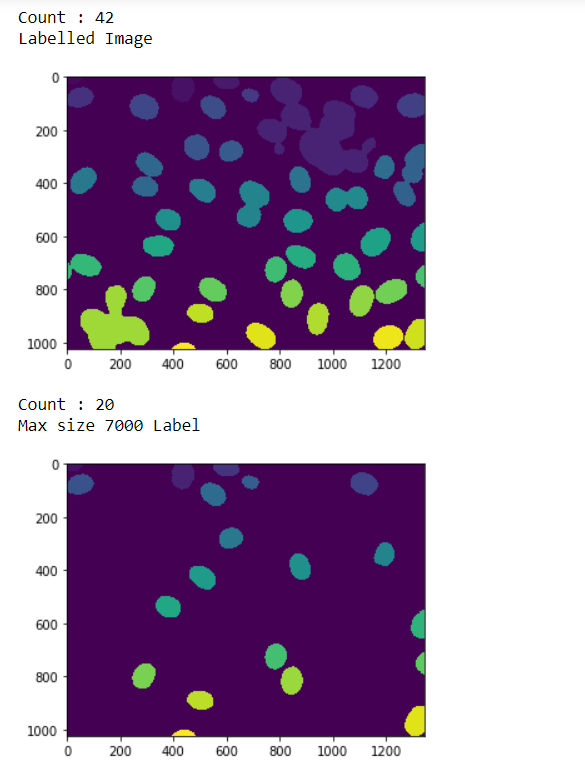# Mahotas – Filtering Labels

In this article we will see how we can filter the labels of the labelled image in mahotas. Filtering label is similar to implementing the relabeling feature but the difference is in filtering we will remove i.e filter the labels at the time of calling the filtering method and filtering will give us new labeled image and number of labels. We use `mahotas.label` method to label the image

For this we are going to use the fluorescent microscopy image from a nuclear segmentation benchmark. We can get the image with the help of command given below

`mhotas.demos.nuclear_image()`

Below is the nuclear_imageLabeled images are integer images where the values correspond to different regions. I.e., region 1 is all of the pixels which have value 1, region two is the pixels with value 2, and so on

In order to do this we will use `mahotas.label.filter_labeled` method

Syntax : mahotas.label.filter_labeled(label_image, filter1, filter2)

Argument : It takes labeled image object and filters as argument

Return : It returns the labelled image and integer i.e number of labels

Note : Filters can be border label filter, maximum size anything.

Example 1:

 `# importing required libraries ` `import` `mahotas ` `import` `numpy as np ` `from` `pylab ``import` `imshow, show ` `import` `os ` ` `  `# loading nuclear image ` `f ``=` `mahotas.demos.load(``'nuclear'``) ` ` `  `# setting filter to the image ` `f ``=` `f[:, :, ``0``] ` ` `  `# setting gaussian filter ` `f ``=` `mahotas.gaussian_filter(f, ``4``) ` ` `  `# setting threshold value ` `f ``=` `(f> f.mean()) ` ` `  `# creating a labeled image ` `labeled, n_nucleus ``=` `mahotas.label(f) ` ` `  `# printing number of labels ` `print``(``"Count : "` `+` `str``(n_nucleus)) ` ` `  `# showing the labeleed image ` `print``(``"Labelled Image"``) ` `imshow(labeled) ` `show() ` ` `  ` `  `# filtering the label image ` `# adding border filter ` `relabeled, n_left ``=` `mahotas.labeled.filter_labeled(labeled, remove_bordering ``=` `True``) ` ` `  `# showing number of labels ` `print``(``"Count : "` `+` `str``(n_left)) ` ` `  `# showing the image ` `print``(``"No border Label"``) ` `imshow(relabeled) ` `show() `

Output :Example 2:

 `# importing required libraries ` `import` `mahotas ` `import` `numpy as np ` `from` `pylab ``import` `imshow, show ` `import` `os ` ` `  `# loading nuclear image ` `f ``=` `mahotas.demos.load(``'nuclear'``) ` ` `  `# setting filter to the image ` `f ``=` `f[:, :, ``0``] ` ` `  `# setting gaussian filter ` `f ``=` `mahotas.gaussian_filter(f, ``4``) ` ` `  `# setting threshold value ` `f ``=` `(f> f.mean()) ` ` `  `# creating a labeled image ` `labeled, n_nucleus ``=` `mahotas.label(f) ` ` `  `# printing number of labels ` `print``(``"Count : "` `+` `str``(n_nucleus)) ` ` `  `# showing the labeleed image ` `print``(``"Labelled Image"``) ` `imshow(labeled) ` `show() ` ` `  ` `  `# filtering the label image ` `# adding max size filter ` `relabeled, n_left ``=` `mahotas.labeled.filter_labeled(labeled, max_size ``=` `7000``) ` ` `  `# showing number of labels ` `print``(``"Count : "` `+` `str``(n_left)) ` ` `  `# showing the image ` `print``(``"Max size 7000 Label"``) ` `imshow(relabeled) ` `show() `

Output :My Personal Notes arrow_drop_upCheck out this Author's contributed articles.

If you like GeeksforGeeks and would like to contribute, you can also write an article using contribute.geeksforgeeks.org or mail your article to contribute@geeksforgeeks.org. See your article appearing on the GeeksforGeeks main page and help other Geeks.

Please Improve this article if you find anything incorrect by clicking on the "Improve Article" button below.

Article Tags :

Be the First to upvote.

Please write to us at contribute@geeksforgeeks.org to report any issue with the above content.